Search by Topic

Resources tagged with Visualising similar to Next Size Up:

Filter by: Content type:
Age range:
Challenge level:

There are 256 results

Broad Topics > Using, Applying and Reasoning about Mathematics > Visualising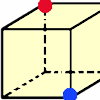Redblue

Age 7 to 11 Challenge Level:

Investigate the number of paths you can take from one vertex to another in these 3D shapes. Is it possible to take an odd number and an even number of paths to the same vertex?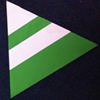Regular Rings 1

Age 7 to 11 Challenge Level:

Can you work out what shape is made by folding in this way? Why not create some patterns using this shape but in different sizes?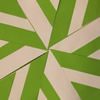Folding Flowers 2

Age 7 to 11 Challenge Level:

Make a flower design using the same shape made out of different sizes of paper.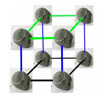More Pebbles

Age 7 to 11 Challenge Level:

Have a go at this 3D extension to the Pebbles problem.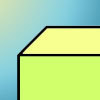Cuboid-in-a-box

Age 7 to 11 Challenge Level:

What is the smallest cuboid that you can put in this box so that you cannot fit another that's the same into it?Inside Seven Squares

Age 7 to 11 Challenge Level:

What is the total area of the four outside triangles which are outlined in red in this arrangement of squares inside each other?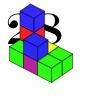28 and It's Upward and Onward

Age 7 to 11 Challenge Level:

Can you find ways of joining cubes together so that 28 faces are visible?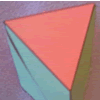Triangular Faces

Age 7 to 11 Challenge Level:

This problem invites you to build 3D shapes using two different triangles. Can you make the shapes from the pictures?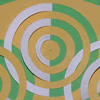Jomista Mat

Age 7 to 11 Challenge Level:

Looking at the picture of this Jomista Mat, can you decribe what you see? Why not try and make one yourself?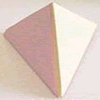Child's Play

Age 7 to 11 Challenge Level:

A toy has a regular tetrahedron, a cube and a base with triangular and square hollows. If you fit a shape into the correct hollow a bell rings. How many times does the bell ring in a complete game?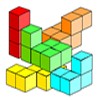Three Cubed

Age 7 to 11 Challenge Level:

Can you make a 3x3 cube with these shapes made from small cubes?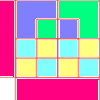Diagrams

Age 7 to 11 Challenge Level:

A group activity using visualisation of squares and triangles.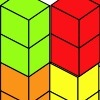Triple Cubes

Age 5 to 11 Challenge Level:

This challenge involves eight three-cube models made from interlocking cubes. Investigate different ways of putting the models together then compare your constructions.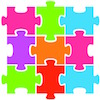Jigsaw Pieces

Age 7 to 11 Challenge Level:

How will you go about finding all the jigsaw pieces that have one peg and one hole?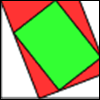Folding, Cutting and Punching

Age 7 to 11 Challenge Level:

Exploring and predicting folding, cutting and punching holes and making spirals.Putting Two and Two Together

Age 7 to 11 Challenge Level:

In how many ways can you fit two of these yellow triangles together? Can you predict the number of ways two blue triangles can be fitted together?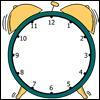A Square in a Circle

Age 7 to 11 Challenge Level:

What shape has Harry drawn on this clock face? Can you find its area? What is the largest number of square tiles that could cover this area?Icosian Game

Age 11 to 14 Challenge Level:

This problem is about investigating whether it is possible to start at one vertex of a platonic solid and visit every other vertex once only returning to the vertex you started at.Triangles to Tetrahedra

Age 11 to 14 Challenge Level:

Imagine you have an unlimited number of four types of triangle. How many different tetrahedra can you make?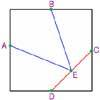Midpoint Triangle

Age 7 to 11 Challenge Level:

Can you cut up a square in the way shown and make the pieces into a triangle?Making Maths: Rolypoly

Age 5 to 11 Challenge Level:

Paint a stripe on a cardboard roll. Can you predict what will happen when it is rolled across a sheet of paper?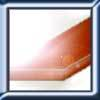Little Boxes

Age 7 to 11 Challenge Level:

How many different cuboids can you make when you use four CDs or DVDs? How about using five, then six?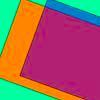Move Those Halves

Age 7 to 11 Challenge Level:

For this task, you'll need an A4 sheet and two A5 transparent sheets. Decide on a way of arranging the A5 sheets on top of the A4 sheet and explore ...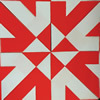Folding Flowers 1

Age 7 to 11 Challenge Level:

Can you visualise what shape this piece of paper will make when it is folded?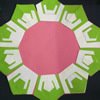Regular Rings 2

Age 7 to 11 Challenge Level:

What shape is made when you fold using this crease pattern? Can you make a ring design?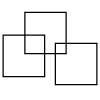Three Squares

Age 5 to 11 Challenge Level:

What is the greatest number of squares you can make by overlapping three squares?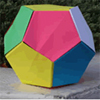Dodecamagic

Age 7 to 11 Challenge Level:

Here you see the front and back views of a dodecahedron. Each vertex has been numbered so that the numbers around each pentagonal face add up to 65. Can you find all the missing numbers?Dicey

Age 7 to 11 Challenge Level:

A game has a special dice with a colour spot on each face. These three pictures show different views of the same dice. What colour is opposite blue?Tetrahedra Tester

Age 11 to 14 Challenge Level:

An irregular tetrahedron is composed of four different triangles. Can such a tetrahedron be constructed where the side lengths are 4, 5, 6, 7, 8 and 9 units of length?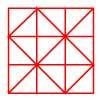Fractional Triangles

Age 7 to 11 Challenge Level:

Use the lines on this figure to show how the square can be divided into 2 halves, 3 thirds, 6 sixths and 9 ninths.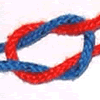Reef and Granny

Age 7 to 11 Challenge Level:

Have a look at what happens when you pull a reef knot and a granny knot tight. Which do you think is best for securing things together? Why?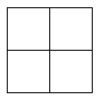Two Squared

Age 7 to 11 Challenge Level:

What happens to the area of a square if you double the length of the sides? Try the same thing with rectangles, diamonds and other shapes. How do the four smaller ones fit into the larger one?Nine Colours

Age 11 to 16 Challenge Level:

Can you use small coloured cubes to make a 3 by 3 by 3 cube so that each face of the bigger cube contains one of each colour?Tetra Square

Age 11 to 14 Challenge Level:

ABCD is a regular tetrahedron and the points P, Q, R and S are the midpoints of the edges AB, BD, CD and CA. Prove that PQRS is a square.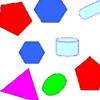Part the Polygons

Age 7 to 11 Short Challenge Level:

Draw three straight lines to separate these shapes into four groups - each group must contain one of each shape.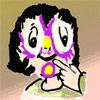Painted Faces

Age 7 to 11 Challenge Level:

Imagine a 3 by 3 by 3 cube made of 9 small cubes. Each face of the large cube is painted a different colour. How many small cubes will have two painted faces? Where are they?Construct-o-straws

Age 7 to 11 Challenge Level:

Make a cube out of straws and have a go at this practical challenge.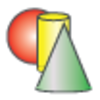Sponge Sections

Age 7 to 11 Challenge Level:

You have been given three shapes made out of sponge: a sphere, a cylinder and a cone. Your challenge is to find out how to cut them to make different shapes for printing.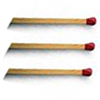Matchsticks

Age 7 to 11 Challenge Level:

Reasoning about the number of matches needed to build squares that share their sides.Sea Defences

Age 7 to 14 Challenge Level:

These are pictures of the sea defences at New Brighton. Can you work out what a basic shape might be in both images of the sea wall and work out a way they might fit together?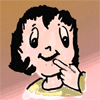Cube Drilling

Age 7 to 11 Challenge Level:

Imagine a 4 by 4 by 4 cube. If you and a friend drill holes in some of the small cubes in the ways described, how many will not have holes drilled through them?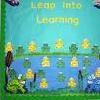Display Boards

Age 7 to 11 Challenge Level:

Design an arrangement of display boards in the school hall which fits the requirements of different people.Pyramid Numbers

Age 7 to 11 Challenge Level:

What are the next three numbers in this sequence? Can you explain why are they called pyramid numbers?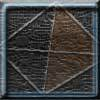Tetrahedron Faces

Age 7 to 11 Challenge Level:

One face of a regular tetrahedron is painted blue and each of the remaining faces are painted using one of the colours red, green or yellow. How many different possibilities are there?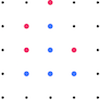Square Surprise

Age 5 to 11 Challenge Level:

Why do you think that the red player chose that particular dot in this game of Seeing Squares?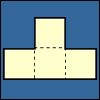Cut Nets

Age 7 to 11 Challenge Level:

Each of the nets of nine solid shapes has been cut into two pieces. Can you see which pieces go together?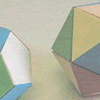Face Painting

Age 7 to 11 Challenge Level:

You want to make each of the 5 Platonic solids and colour the faces so that, in every case, no two faces which meet along an edge have the same colour.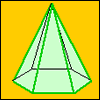A Chain of Eight Polyhedra

Age 7 to 11 Challenge Level:

Can you arrange the shapes in a chain so that each one shares a face (or faces) that are the same shape as the one that follows it?World of Tan 13 - A Storm in a Tea Cup

Age 7 to 11 Challenge Level:

Can you fit the tangram pieces into the outlines of the convex shapes?Bands and Bridges: Bringing Topology Back

Age 7 to 14

Lyndon Baker describes how the Mobius strip and Euler's law can introduce pupils to the idea of topology.